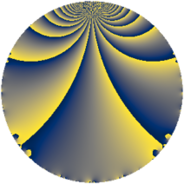# Properties

 Label 71.1.bLevel $71$ Weight $1$ Character orbit 71.b Rep. character $\chi_{71}(70,\cdot)$ Character field $\Q$ Dimension $3$ Newform subspaces $1$ Sturm bound $6$ Trace bound $0$

# Related objects

## Defining parameters

 Level: $$N$$ $$=$$ $$71$$ Weight: $$k$$ $$=$$ $$1$$ Character orbit: $$[\chi]$$ $$=$$ 71.b (of order $$2$$ and degree $$1$$) Character conductor: $$\operatorname{cond}(\chi)$$ $$=$$ $$71$$ Character field: $$\Q$$ Newform subspaces: $$1$$ Sturm bound: $$6$$ Trace bound: $$0$$

## Dimensions

The following table gives the dimensions of various subspaces of $$M_{1}(71, [\chi])$$.

Total New Old
Modular forms 4 4 0
Cusp forms 3 3 0
Eisenstein series 1 1 0

The following table gives the dimensions of subspaces with specified projective image type.

$$D_n$$ $$A_4$$ $$S_4$$ $$A_5$$
Dimension 3 0 0 0

## Trace form

 $$3 q - q^{2} - q^{3} + 2 q^{4} - q^{5} - 2 q^{6} - 2 q^{8} + 2 q^{9} + O(q^{10})$$ $$3 q - q^{2} - q^{3} + 2 q^{4} - q^{5} - 2 q^{6} - 2 q^{8} + 2 q^{9} - 2 q^{10} - 3 q^{12} - 2 q^{15} + q^{16} + 4 q^{18} - q^{19} + 4 q^{20} + 3 q^{24} + 2 q^{25} - 2 q^{27} - q^{29} + 3 q^{30} - 3 q^{32} - q^{36} - q^{37} + 5 q^{38} - 4 q^{40} - q^{43} - 3 q^{45} + 2 q^{48} + 3 q^{49} - 3 q^{50} - 4 q^{54} - 2 q^{57} - 2 q^{58} + q^{60} + 3 q^{71} + q^{72} - q^{73} + 5 q^{74} + 4 q^{75} - 3 q^{76} - q^{79} + 2 q^{80} + q^{81} - q^{83} - 2 q^{86} + 5 q^{87} - q^{89} + q^{90} - 2 q^{95} + q^{96} - q^{98} + O(q^{100})$$

## Decomposition of $$S_{1}^{\mathrm{new}}(71, [\chi])$$ into newform subspaces

Label Dim $A$ Field Image CM RM Traces $q$-expansion
$a_{2}$ $a_{3}$ $a_{5}$ $a_{7}$
71.1.b.a $3$ $0.035$ $$\Q(\zeta_{14})^+$$ $D_{7}$ $$\Q(\sqrt{-71})$$ None $$-1$$ $$-1$$ $$-1$$ $$0$$ $$q-\beta _{1}q^{2}+(-1+\beta _{1}-\beta _{2})q^{3}+(1+\beta _{2})q^{4}+\cdots$$